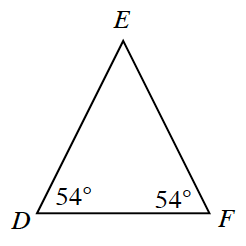Home > CCG > Chapter 5 > Lesson 5.3.2 > Problem5-89

5-89.

Lizzie noticed that two angles in $ΔDEF$, shown below, have the same measure. Based on this information, what statement can you make about the relationship between $\overline{DE}$ and $\overline{EF}$? Homework Help ✎They must have equal lengths, because if base angles are equal, then the sides opposite them are equal and the triangle is isosceles.